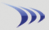Subscription & data-feed pricing Class schedule

Margin rates Stock & option commissions

Attention: Discussion forums are read-only for extended maintenance until further notice.

 R3 Strategy Rate this Topic:Previous Topic · Next Topic Watch this topic · Print this topic ·
couto7
 Posted : Tuesday, May 29, 2018 11:58:29 AMGold Customer

Joined: 10/14/2004
Posts: 202

Please write PCF with the following parameters:

1. Price above the 200 day MA (simple)                                                                                                        2. The 2-period RSi drops three days in a row and the first day's drop is from below 60                              3. The 2-period RSI closes under 10 on third day which is the day of the trade (buy signal)

4.  Exit when the 2-period RSI closes above 70

-Thanks

couto7
 Posted : Tuesday, May 29, 2018 12:05:57 PMGold Customer

Joined: 10/14/2004
Posts: 202

Bruce,

Sorry for earlier post with confusing layout.

Price above the 200 simple day MA and the RSI drops three days in a row and first day's drop is from below 60.  The RSI closes under 10 on third day, the day which trade is triggered.

Exit when the 2-period RSI closes above 70.

Thanks

Bruce_L
 Posted : Tuesday, May 29, 2018 12:22:10 PMWorden Trainer

Joined: 10/7/2004
Posts: 65,138

If Use Wilder's Smoothing is not checked.

`C > AVGC200 AND RSI14 < 10 AND RSI2 < RSI2.1.1 AND RSI2.1.1 < RSI2.1.2 AND RSI2.1.2 < RSI2.1.3 AND RSI2.1.3 < 60`

Exit:

`RSI2 > 70`

If Use Wilder's Smoothing is checked, the formulas will be quite a bit longer.

`C > AVGC200 AND C < C1 AND C1 < C2 AND C2 < C3 AND 50 * (C - XAVGC3.1) / 2 / (.50003052 * (ABS(C - C1) + .5 * (ABS(C1 - C2) + .5 * (ABS(C2 - C3) + .5 * (ABS(C3 - C4) + .5 * (ABS(C4 - C5) + .5 * (ABS(C5 - C6) + .5 * (ABS(C6 - C7) + .5 * (ABS(C7 - C8) + .5 * (ABS(C8 - C9) + .5 * (ABS(C9 - C10) + .5 * (ABS(C10 - C11) + .5 * (ABS(C11 - C12) + .5 * (ABS(C12 - C13) + .5 * (ABS(C13 - C14)))))))))))))))) + 50 < 10 AND 50 * (C3 - XAVGC3.4) / 2 / (.50003052 * (ABS(C3 - C4) + .5 * (ABS(C4 - C5) + .5 * (ABS(C5 - C6) + .5 * (ABS(C6 - C7) + .5 * (ABS(C7 - C8) + .5 * (ABS(C8 - C9) + .5 * (ABS(C9 - C10) + .5 * (ABS(C10 - C11) + .5 * (ABS(C11 - C12) + .5 * (ABS(C12 - C13) + .5 * (ABS(C13 - C14) + .5 * (ABS(C14 - C15) + .5 * (ABS(C15 - C16) + .5 * (ABS(C16 - C17)))))))))))))))) + 50 < 60`

Exit:

`50 * (C - XAVGC3.1) / 2 / (.50003052 * (ABS(C - C1) + .5 * (ABS(C1 - C2) + .5 * (ABS(C2 - C3) + .5 * (ABS(C3 - C4) + .5 * (ABS(C4 - C5) + .5 * (ABS(C5 - C6) + .5 * (ABS(C6 - C7) + .5 * (ABS(C7 - C8) + .5 * (ABS(C8 - C9) + .5 * (ABS(C9 - C10) + .5 * (ABS(C10 - C11) + .5 * (ABS(C11 - C12) + .5 * (ABS(C12 - C13) + .5 * (ABS(C13 - C14)))))))))))))))) + 50 > 70`

-Bruce
Personal Criteria Formulas
TC2000 Support Articles
Users browsing this topic
Guest-1

 Forum Jump Customer Training & Support - Ask a Trainer - TC2000 version 12/18 - Ask a Trainer - TC2000 version 7 - Ask a Trainer - StockFinder 5.0 - PCFs, EasyScan and Custom Indicators General Discussions - Stock and Market Talk - TC2000 version 12 or 18 - TC2000 version 7 - StockFinder 5.0 - RealCode for StockFinder 5.0 Tutorial Videos - TC2000 version 12 tutorial videos - TC2000 version 7 tutorial videos You cannot post new topics in this forum. You cannot reply to topics in this forum. You cannot delete your posts in this forum. You cannot edit your posts in this forum. You cannot create polls in this forum. You cannot vote in polls in this forum.